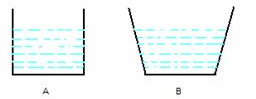Courses

# Test: Pressure in Fluids

## 10 Questions MCQ Test Physics Class 11 | Test: Pressure in Fluids

Description
This mock test of Test: Pressure in Fluids for JEE helps you for every JEE entrance exam. This contains 10 Multiple Choice Questions for JEE Test: Pressure in Fluids (mcq) to study with solutions a complete question bank. The solved questions answers in this Test: Pressure in Fluids quiz give you a good mix of easy questions and tough questions. JEE students definitely take this Test: Pressure in Fluids exercise for a better result in the exam. You can find other Test: Pressure in Fluids extra questions, long questions & short questions for JEE on EduRev as well by searching above.
QUESTION: 1

### The pressure of 1 atm = ______ Pa

Solution:

Pressure of 1 atm = 1.013 x 105 Pa

QUESTION: 2

### Which of the following is not an application of Pascal’s Law?

Solution:

Pascal's law states that the magnitude of pressure within the fluid is equal in all parts.

Option A: Brahma's press is a Hydraulic Press that works on the principle of Pascal's law.

Option B: Submarines don't work on the principle of Pascal's law.

Option C: Hydraulic lifts works on the principle of pascals law i.e. a force applied on a smaller cylinder is transmitted to lift heavy loads using larger cylinders.

Hence, option B is correct.

QUESTION: 3

### In a car lift, compressed air exerts a force F1​ on a small piston having a radius of 5cm. This pressure is transmitted to the second piston of a radius of 15cm. If the mass of the car to be lifted is 1350 kg. What is F1​?

Solution:

From Pascal’s law: P​= P2

⇒ F1/A​​= ​F2/A2​​
⇒ F1/πr1​2​ ​= F2/πr2​​
⇒ F1 = (F* r1​2 ) / r22  = 1350 * 9.8 * (5 * 10-2)2 / (15 * 10-2)2 = 1470 N ​= 1.47 * 10N

QUESTION: 4

What is torr?

Solution:

The torr is a unit of pressure based on an absolute scale, defined as exactly 1/760 of a standard atmosphere.
It is equivalent to 1 mm of mercury in a barometer and equal to 133.32 pascals.

QUESTION: 5

The formula used to find the pressure on a swimmer h meters below the surface of a lake is: (where Pa is the atmospheric pressure)

Solution:

We know that the pressure at some point inside the water can be represented by: Pa + ρhg
where,
ρ = Density of the liquid
Pa = Atmospheric pressure
H = Depth at which the body is present
g = Gravitational acceleration

QUESTION: 6

The pressure at the bottom of a tank containing a liquid does not depend on:

Solution:

Pressure at the bottom of a tank containing liquid is given as P = hρg which is independent of the surface area bottom of the tank.

QUESTION: 7

Find the density when a liquid 5 m high in a column exerts a pressure of 80 Pa.

Solution:

► Pressure = Density x Gravity x Height = ρgh
⇒  ρ = P/(g*h) = 80 Pa / (9.8 m/s2 x 5 m)
Density = 1.632 kg/m3

QUESTION: 8

Two vessels with equal base and unequal height have water filled to the same height. The force at the base of the vessels is:Solution:

Force at the bases of two vessels will be equal as force depends on height and area. Since it is the same here, force is equal.

QUESTION: 9

Which of the following devices in not based on Pascal’s law?

Solution:
• Atomizers work on the principle of airflow and suction i.e. on Bernoulli's principle and not on Pascal's law.
• On the other hand, Syringe, hydraulic brakes and hydraulic lift work on the principle of Pascal's law.
QUESTION: 10

The pressure measured by a gauge is:

Solution:

Gauge pressure is the pressure relative to atmospheric pressure. Gauge pressure is positive for pressures above atmospheric pressure and negative for pressures below it.

Pabs = P+ Patm
P= Pabs - Patm